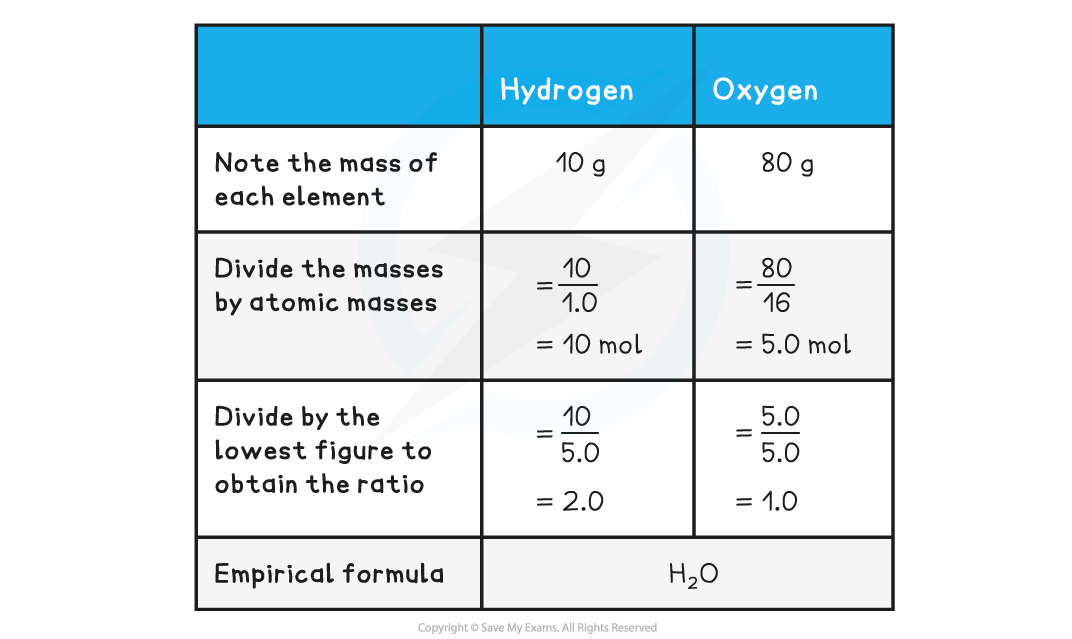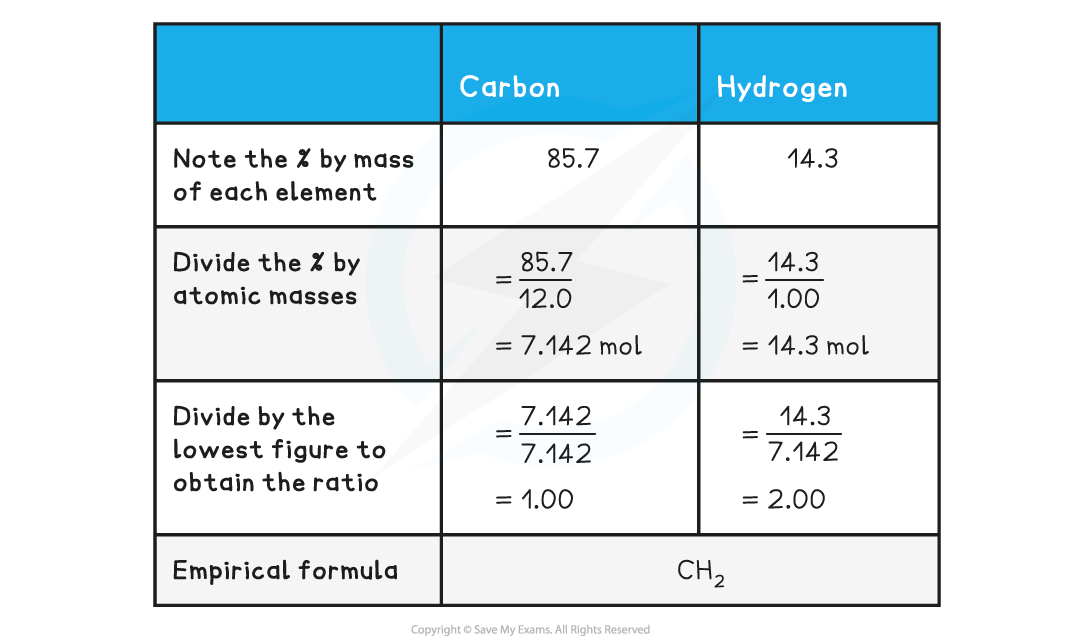# AQA A Level Chemistry复习笔记1.2.2 Empirical & Molecular Formula

### Empirical & Molecular Formulae

• The molecular formula is the formula that shows the number and type of each atom in a molecule
• E.g. the molecular formula of ethanoic acid is C2H4O2

• The empirical formula is the simplest whole number ratio of atoms of each element present in one molecule or formula unit of a compound
• E.g. the empirical formula of ethanoic acid is CH2O

### Empirical & Molecular Formulae Calculations

#### Empirical formula

• Empirical formula is the simplest whole number ratio of the elements present in one molecule or formula unit of the compound
• It is calculated from knowledge of the ratio of masses of each element in the compound
• The empirical formula can be found by determining the mass of each element present in a sample of the compound
• It can also be deduced from data that gives the percentage compositions by mass of the elements in a compound

#### Worked Example

Empirical formula from mass

Determine the empirical formula of a compound that contains 10 g of hydrogen and 80 g of oxygen.• The above example shows how to calculate empirical formula from the mass of each element present in the compound
• The example below shows how to calculate the empirical formula from percentage composition

#### Worked Example

Empirical formula from %

Determine the empirical formula of a compound that contains 85.7% carbon and 14.3% hydrogen.#### Molecular formula

• The molecular formula gives the exact numbers of atoms of each element present in the formula of the compound
• The molecular formula can be found by dividing the relative formula mass of the molecular formula by the relative formula mass of the empirical formula
• Multiply the number of each element present in the empirical formula by this number to find the molecular formula

#### Worked Example

Calculating molecular formula

The empirical formula of X is C4H10S and the relative molecular mass of X is 180
What is the molecular formula of X?

(Ar data: C = 12, H = 1, S = 32)

Step 1: Calculate relative mass of the empirical formula

• Relative empirical mass = (C x 4) + (H x 10) + (S x 1)
• Relative empirical mass = (12 x 4) + (1 x 10) + (32 x 1)
• Relative empirical mass = 90

Step 2: Divide relative formula mass of X by relative empirical mass

• Ratio between Mr of X and the Mr of the empirical formula = 180/90
• Ratio between Mr of X and the Mr of the empirical formula = 2

Step 3: Multiply each number of elements by 2

• (C4 x 2) + (H10 x 2) + (S x 2)     =    (C8) + (H20) + (S2)
• Molecular Formula of X is C8H20S2